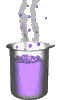Science class online
Chemistry and physics lessons

# Electricity

Free online electricity lessons for elementary school,  middle school and high school.Science class Chemistry Electricity Optics Mechanics Electricity lessons Electrical components -Two-terminal electronic components - Basic electrical components - Diodes - What's a resistor ? - How to determine a resistor value ? - Resistors effects in circuits - How to use a resistor ? - Characteristic curve of a resistor How to build simple circuits and draw diagrams - How to build a basic electrical circuit - How to draw diagrams of electric circuits The electric current - Conductors and insulators - Direction of electric current in a circuit - The dangers of electricity - Current intensity - units how to measure current ? The Voltage - The voltage and its units - How to measure a voltage ? - Voltage in open and closed circuits - Rated current and voltage for a lamp Alternating voltage and current - Alternating currents effects on led - What are alternating current and voltage ? - Periodic alternating voltage and its properties - Oscilloscope - Oscillogram - Frequency - Measuring RMS voltage with a voltmeter Serie circuits - What's a serie circuit ? - Series circuit properties - Short circuit in  series circuit - Current law in series - circuits - Voltage law in series circuits Parallel circuits - What's a parallel circuit ? - Some parallel circuits properties - Short circuits in parallel circuits - Nodes and branches in parallel circuits - Current laws in parallel circuits - Voltage laws in parallel circuits Laws of electricity - Ohm's law - Current laws in series circuits - Voltage laws in series circuits - Current laws in parallel circuits - Voltage laws in parallel circuits Generating electricity - Voltage for coil wires What is an alternator ? - Alternating currents and voltages - Generating electricity in power plants Electric power and energy - Electric power and power rating - Electric power consumption by an electrical device - Relationship between - Electric power and energy

 _____________________________________________________   _____________________________________________________

## Alternative currents and voltages

### What are alternating currents and voltages ?

 1) Direct voltages and currents A DC voltage generator (such as a battery) provides a constant voltage in value but also in sign. A DC voltage generator produces a current that always flows in the same direction (from negative terminal to positive terminal ) 2) Alternative voltages An alternator is a generator that provides an alternating voltage and an alternating current. When a generator operates its magnet (or electromagnet) is rotating: the magnetic north pole approaches and then moves away from the coil and then it is the turn the south pole. This succession of approaches and moving away of the two poles causes a succession of positive and negative voltage at the terminals of coil. The voltage supplied by a alternator is called alternative because it is alternately positive and negative. ______________________________________ ______________________________________ 3) Alternating currents An alternator's voltage is alternately positive and negative ​​because of its terminals that also change sign. The electric current that flows from negative terminal to positive terminal changes its direction when these terminals change their sign: The current flows alternately in one direction and then in another. ______________________________________ ______________________________________
____________________________________

____________________________________